Courses

# Competition Level Test: Dual Nature of Radiation and Matter

## 26 Questions MCQ Test Physics For JEE | Competition Level Test: Dual Nature of Radiation and Matter

Description
This mock test of Competition Level Test: Dual Nature of Radiation and Matter for Class 12 helps you for every Class 12 entrance exam. This contains 26 Multiple Choice Questions for Class 12 Competition Level Test: Dual Nature of Radiation and Matter (mcq) to study with solutions a complete question bank. The solved questions answers in this Competition Level Test: Dual Nature of Radiation and Matter quiz give you a good mix of easy questions and tough questions. Class 12 students definitely take this Competition Level Test: Dual Nature of Radiation and Matter exercise for a better result in the exam. You can find other Competition Level Test: Dual Nature of Radiation and Matter extra questions, long questions & short questions for Class 12 on EduRev as well by searching above.
QUESTION: 1

### In a photo-emissive cell, with exciting wavelength l, the maximum kinetic energy of electron is K. If the exciting wavelength is changed to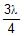the kinetic energy of the fastest emitted electron will be :

Solution:

From E=W0​+(1/2)​mvmax2​⇒vmax​= √[(2E/m)​−(2W0/m)]​​​
where E= hc​/λ
If wavelength of incident light charges from λ to 3λ/4​ (Decreases)
Let energy of incident light charges from E and speed of fastest electron changes from v to v′ then
v′=√[(2E′/m)​−(2W0/m)​​​]
As E∝1/λ​⇒E′(4/3)​E
Hence v′=√[(2(4/3​)E/m)​−(2W0/m)​​]​
⇒v′=(4/3)1/2 [(2E/m)​− (2W0/m(4/3)1/2​)]​​
So, v′>(4/3)1/2v

QUESTION: 2

### The frequency and the intensity of a beam of light falling on the surface of photoelectric material are increased by a factor of two (Treating efficiency of photoelectron generation as constant). This will :

Solution: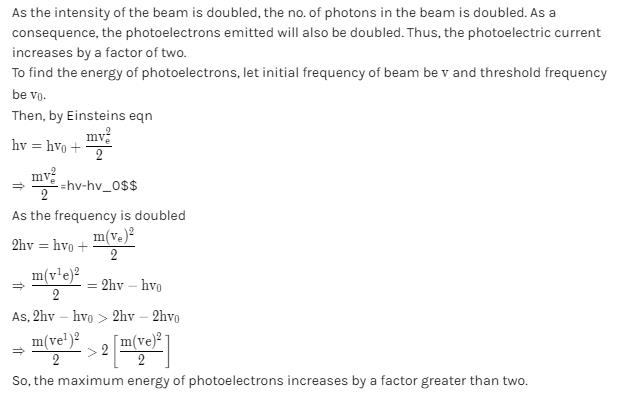QUESTION: 3

### Light coming from a discharge tube filled with hydrogen falls on the cathode of the photoelectric cell. The work function of the surface of cathode is 4eV. Which one of the following values of the anode voltage (in Volts) with respect to the cathode will likely to make the photo current zero.

Solution:

Given,
Work function, ϕ=4eV
for Hydrogen atom, it can provide a max of 13.6 eV of energy by exciting its electron.
∴ e(Stopping potential) ≥ 13.6 - 4
≥ 9.6
i.e. V = 10 V will make photo current zero.

QUESTION: 4

Let K1 be the maximum kinetic energy of photoelectrons emitted by a light of wavelength l1 and K2 corresponding to l2. If l1 = 2l2, then :

Solution:

We know that,
[(hc/ λ) -Wo]=K1 =>l1=hc/(K1 +Wo)
[(hc/l2 )-Wo]=K2=>l2= hc/(K2+ Wo)
Given, l1=2l2
=> hc/K2+ Wo =2hc/(K2+ Wo)
Or,K1+Wo= (K2+ Wo)/2
Or,K1=(K2/2)-(Wo/2)
Since, Wo >1=>K1<K2/2

QUESTION: 5

In a photoelectric experiment, the potential difference V that must be maintained between the illuminated surface and the collector so as just to prevent any electron from reaching the collector is determined for different frequencies f of the incident illumination. The graph obtained is shown. The maximum kinetic energy of the electrons emitted at frequency f1 is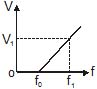Solution: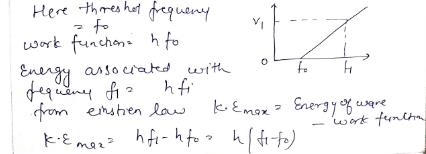*Multiple options can be correct
QUESTION: 6

In photoelectric effect, stopping potential depends on

Solution:

The stopping potential depends on frequency of incident light and nature of the emitter material.for a given frequency of incident light, it is independent of its intensity.

*Multiple options can be correct
QUESTION: 7

In the experiment on photoelectric effect using light having frequency greater than the threshold frequency, the photocurrent will certainly increase when

Solution:

If light of certain frequency, greater than the threshold frequency, is incident of the metal surface the photoelectric current obtained is directly proportional to the number of photo-electrons emitted. On increasing the frequency of incident light (or) by increasing the anode potential the speed of photo-electrons emitted changes but they have no effect on the number of electrons emitted. But by increasing the intensity of the incident light more photo-electrons will be emitted and thereby increasing the photoelectric current.
Also, if we increase the area on which the light is incident we can get more electrons emitted which increases the photoelectric current.

QUESTION: 8

Two electrons are moving with the same speed v. One electron enters a region of uniform electric field while the other enters a region of uniform magnetic field, then after sometime if the de-Broglie wavelengths of the two are l1 and l2 then :

Solution:
QUESTION: 9

An electron in hydrogen atom first jumps from second excited state to first excited state and then, from first excited state to ground state. Let the ratio of wavelength, momentum and energy of photons in the two cases by x, y and z, then select the wrong answers :

Solution:
• 1/λ=R[(1/nf2)-(1/nl2)
• Therefore, for 3->2 , we get,
• 1/λ1=R[(1/22)-(1/32)]=R[(1/4)-(1/9)]=5R/36
• Therefore, λ1=36/5R
• Similarly, for 2->1, we get,
• 1/λ2=R[(1/12)-(1/22)]=R[(1-(1/4)]=3R/4
• Therefore, λ2=4/3R
• Therefore, the ratio is,
• x=λ12=(36/5R) x (3R/4)=27/5
• hence, option B is wrong.
•
• Now, the momentum is given as,
• p=h/λ
• Therefore, 3->2
• p1=h/λ1=5Rh/36
• similarly, for 2->1, we get,
• p2 =h/λ2=3Rh/4
• therefore, the ratio is,
• y=p1/p2=(5Rh/36)x(4/3Rh)=5/27
• hence, option C is correct.
• Now the energy difference between the two levels is,
• ΔE=En-1-En
• Therefore, for 3->2
• ΔE1=E2-E3=(E1/22)-(E1/32)=E1[(1/4)-(1/9)]=5E1/36
• Similarly, 2->1
• ΔE2=E1-E2=E1-(E1/22)=E1[1-(1/4)]=3E1/4
• Therefore, the ratio is,
• z= ΔE1/ΔE2=(5E1/36) X (4/3E1)=5/27
• hence, option A and D are both correct.
• therefore, we can say that the wrong is option (B)

QUESTION: 10

An electron is in an excited state in hydrogen-like atom. It has a total energy of –3.4 eV. If the kinetic energy of the electron is E and its de-Broglie wavelength is l, then

Solution:

The potential energy of an electron is twice the kinetic energy(with negative sign) of an electron.
PE=2E
The total energy is: TE=PE+KE
−3.4=−2×3.4+KE
KE=3.4eV
Let p be the momentum of an electron and m be the mass of an electron.
E=p2​/2m
p=√2​mE

Now, the De-Broglie wavelength associated with an electron is:
λ=h/p​
λ=h/√2​mE​
λ=6.6×1034​/√2​×9.1×10−31×(−3.4)×1.6×10−19
λ=6.6×1034​/9.95×10−25
0λ=0.66×10−9
λ=6.6×10−10m

*Multiple options can be correct
QUESTION: 11

A particular hydrogen like atom has its ground state binding "energy 122.4 eV. Its is in ground state. Then :

Solution:

En​= −z213.6eV/ n2
for ground state, n=1
−122.4=−Z213.6eV
When a proton interacts that
EP​=hV=E1​−E0​=Z213.6eV[(1/12​)−(1/22)​]
=9×13.6eV [3/4]
=91.8eV
So the Atomic number is 3 , a photon  of 91.8eV and an electron of 8.2eV are emitted when 100eV electron interacts e− interact with this atom.

*Multiple options can be correct
QUESTION: 12

The electron in a hydrogen atom makes a transition n1 ¾→ n2, where n1 & n2 are the principal quantum numbers of the two states. Assume the Bohr model to be valid. The time period of the electron in the initial state is eight times that in the final state. The possible values of n1 & n2 are :

Solution:

For an electron revolving in nth orbit around the nucleus of hydrogen atom, Fcentrifugal​=Fcoulomb​
Thus,  mvn2/ rn​​​=​e2​/4πϵo​rn2
Also, mvn​rn​=nh/2π​
On solving these two we get: vn​= e21/2ϵo​hn ​and rn​=( ϵo​h2/πme2 ​) n2
Time period, T=2πrn/vn​​​⟹T∝n3
Thus, 8T/T​=(​n1/n2)3⟹n1​=2n2
Thus, n1​=4,n2​=2  and   n1​=6,n2​=3

*Multiple options can be correct
QUESTION: 13

A beam of ultraviolet light of all wavelengths passes through hydrogen gas at room temperature, in the x-direction. Assume that all photons emitted due to electron transition inside the gas emerge in the y-direction. Let A and B denote the lights emerging from the gas in the x and y directions respectively.

Solution:

Given, incident wavelengths travel in x-direction which are in the U.V region, which means they can excite an electron from n=1 to higher levels. Hence, some of the incident wavelengths will be absent in A.
Photons emerging due to transitions of electrons to their ground state contains visible region wavelengths if transition is done into n=2. If transition is done into n>2, photons contain I.R wavelengths.
Therefore, A,C,D is the correct choice.

*Multiple options can be correct
QUESTION: 14

If radiation of allow wavelengths from ultraviolet to infrared is passed through hydrogen agas at room temperature, absorption lines will be observed in the :

Solution:

At room temperature, nearly all the atoms in hydrogen gas will be in the ground state. When light passes through the gas, photons are absorbed, causing electrons to make transitions to higher states and creating absorption lines. These lines correspond to the Lyman series since that is the series of transitions involving the ground state or n = 1 level. Since there are virtually no atoms in higher energy states, photons corresponding to transitions from n > 2 to higher states will not be absorbed.

QUESTION: 15

In the hydrogen atom, if the reference level of potential energy is assumed to be zero at the ground state level. Choose the incorrect statement.

Solution:

Explanation : When the electron changes levels, it decreases energy and the atom emits photons. The photon is emitted with the electron moving from a higher energy level to a lower energy level. The energy of the photon is the exact energy that is lost by the electron moving to its lower energy level.

*Multiple options can be correct
QUESTION: 16

Statement-1: Figure shows graph of stopping potential and frequency of incident light in photoelectric effect. For values of frequency less than threshold frequecy (v0) stopping potential is negative.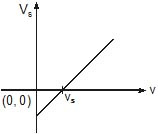Statement-2 : Lower the value of frequency of incident light (for n > n0) the lower is the maxima of kinetic energy of emitted photoelectrons.

Solution:
QUESTION: 17

Statement-1: In the process of photo electric emission, all the emitted photoelectrons have same K.E.

Statement-2: According to einstein's photo electric equation KEmax = hn – f.

Solution:

Explanation : a) Metals emit electrons when light shines upon them. The phenomenon is named as photoelectric effect and the electrons emitted in such manner are called photoelectrons.

The kinetic energy of photoelectrons vary simply because of the fact that (after photoelectric effect has taken place) all the photoelectrons are not emitted instantly. Most of the photoelectrons first collide (several times!) with the other electrons (which are roaming around with random velocities; recall that metals are generally electrically conductive i.e. having abundant free electrons) within the metal. These photoelectrons lose some fraction of their kinetic energy in such collisions before they are emitted out of the metal.

That's why it is observed that the photoelectrons do not have the same kinetic energy.

b) In the photoelectric effect, each photon donates all of its energy hf to an electron in the metal. If this process occurs at the metal surface, the electron is released into the vacuum with a kinetic energy given by:

Kmax = hf - phi

*Multiple options can be correct
QUESTION: 18

Statement-1: Work function of aluminum is 4.2 eV. If two photons each of energy 2.5 eV strikes on a piece of aluminum, the photo electric emission does not occur.

Statement-2: In photo electric effect a single photon interacts with a single electron and electron is emitted only if energy of each incident photon is greater then the work function.

Solution:
*Multiple options can be correct
QUESTION: 19

Statement-1: An electron and a proton are accelerated through the same potential difference. The deBroglie wavelength associated with the electron is longer.

Statement-2: De-Broglie wavelength associated with a moving particle is l =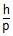where, p is the linear momentum and both have same K.E.

Solution:

de Broglie Wavelength for Electrons
In the case of electrons going in circles around the nuclei in atoms, the de Broglie waves exist as a closed-loop, such that they can exist only as standing waves, and fit evenly around the loop. Because of this requirement, the electrons in atoms circle the nucleus in particular configurations, or states, which are called stationary orbits.
de Broglie Wavelength Formula and Derivation
de Broglie reasoned that matter also can show wave-particle duality, just like light, since light can behave both as a wave (it can be diffracted and it has a wavelength) and as a particle (it contains packets of energy hν). And also reasoned that matter would follow the same equation for wavelength as light namely,
λ = h / p
Where p is the linear momentum, as shown by Einstein.
Derivation
de Broglie derived the above relationship as follows:
1) E = hν for a photon and λν = c for an electromagnetic wave.
2) E = mc2, means λ = h/mc, which is equivalent to λ = h/p.
Note: m is the relativistic mass, and not the rest mass; since the rest mass of a photon is zero.
Now, if a particle is moving with a velocity v, the momentum p = mv and hence λ = h / mv
Therefore, the de Broglie wavelength formula is expressed as;
λ = h / mv

QUESTION: 20

Statement-1: Two photons having equal wavelengths have equal linear momenta.

Statement-2: When light shows its photon character, each photon has a linear momentum p = .

Solution:

Explanation : Statement I is incorrect

The correct statement is : Two photons having Equal linear momenta have equal wavelengths.

*Answer can only contain numeric values
QUESTION: 21

A parallel beam of uniform, monochromatic light of wavelength 2640 A has an intensity of 200 W/m2. The number of photons in 1 mm3 of this radiation are ...............

Solution:

I=nhv/ΔtA=[N.h(C/ λ)]/(1mm/c)x(1mm2)
N=200x10-9x2640x10-10/(3x108)2x6.63x10-34
=885 Photons/mm3

QUESTION: 22

The total energy of the electron in the hydrogen atom in the ground state is -13.6 eV. Which of the following is its kinetic energy in the first excited state?

Solution:

We know that,
∣P.E∣/2​=∣T.E∣=K.E
So, ∣P.E∣/2​=3.4=K.E
So, kinetic energy    K.E=3.4 eV

*Answer can only contain numeric values
QUESTION: 23

When a monochromatic point source of light is at a distance of 0.2 m from a photoelectric cell, the cut off voltage and the saturation current are respectively 0.6 volt and 18.0 mA. If the same source is placed 0.6 m away from the photoelectric cell, then find

Q

the stopping potential

Solution:

Intensity of photons ↑, no. of electron ↑

The cut off voltage does not depend on distance between cell and source.
But intensity is inversely proportional to square of it's distance.
∴ at d=0.6m,
Cut off voltage =0.6V

but
Is​=18×(0.2/0.6 ​)2
=2mA

*Answer can only contain numeric values
QUESTION: 24

When a monochromatic point source of light is at a distance of 0.2 m from a photoelectric cell, the cut off voltage and the saturation current are respectively 0.6 volt and 18.0 mA. If the same source is placed 0.6 m away from the photoelectric cell, then find

Q.

the saturation current

Solution:

Intensity of photons ↑, no. of electron ↑
The cut off voltage does not depend on distance between cell and source.
But intensity is inversely proportional to square of it's distance.
∴ at d=0.6m,
Cut off voltage =0.6V
but
Is​=18×(0.2/0.6​)2
=2mA
So, the answers are option 2.0 mA

*Answer can only contain numeric values
QUESTION: 25

An isolated metal body is illuminated with monochromatic light and is observed to become charged to a steady positive potential 1.0 V with respect to the surrounding. The work function of the metal is 3.0 eV. The frequency of the incident light is ___________________ eV.

Solution:

By Einstein's Photoelectric equation
eV=hν−ϕ
where V=1V= cut off voltage
⟹ν= (eV+ϕ)/ h ​=4eV/h​=4×1.6×10−19​/6.63×10−34
⟹ν=9.6×1014Hz

QUESTION: 26

663 mW of light from a 540 nm source is incident on the surface of a metal. If only 1 of each 5×109 incident photons in absorbed and causes an electron to be ejected from the surface, the total photocurrent in the circuit is ____________________.

Solution:

N / Δt = no. of photon incident per second.
∴ 663x10-3=(N/Δt)x(hc/λ)
∴N/ Δt=663x10-3/(hc/λ)= 663x10-3/(1242nmeV/540)
(n/Δt)/(N/Δt)=1/(5x109)
n/Δt=[1/(5x109)]x (N/Δt)
=[1/(5x109)]x ((663x10-3x540)/1242nmeV)
I=ne/Δt=5.76x1011A